# Constraints Tool - Complete Example¶

## RSM Multiple Linear Constraints Tool¶

### Example #1:¶

1. Define the boundaries using “Optimal” design: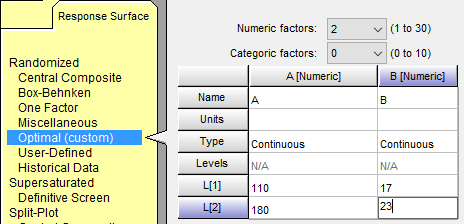The need for a constraint arises because low A and low B at the same time will not work. At the lowest level of A factor B must be at least 19. At the lowest level of B factor A must be at least 180.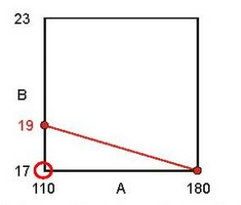1. Press the “Edit Constraints” button. Enter the inequalities that define the region of operability. Click the “Constraint Tool” if you would like assistance writing the inequalities.

1. Select the vertex being constrained (excluded) from the design. For this example it is the low, low combination where A = 110 and B = 17. Change A from 180 to 110, after you do this the “A <” constraint will change to “A >”. Change B from 23 to 17, after you do this the “B <” constraint will change to “B >”.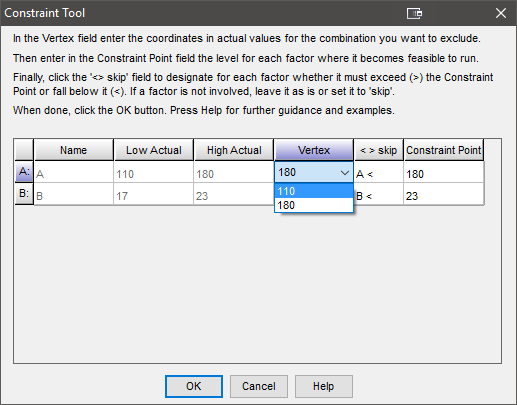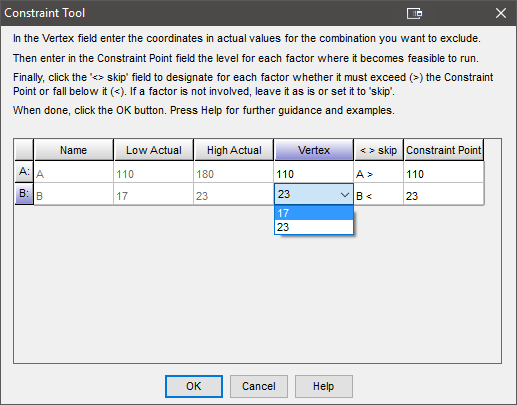1. To define the constraint points, answer the following question for each factor at the excluded vertex. “What are the acceptable values for a given factor when all other factors are forced to the excluded vertex values?”

For this example two statements are necessary to define the constraint:
1. When B is 17, A must be at least 180; make sure the constraint type is “A >” and enter 180 in the constraint point for A.

2. When A is 110, B must be at least 19; make sure the constraint type is “B >” and enter 19 in the constraint point for B.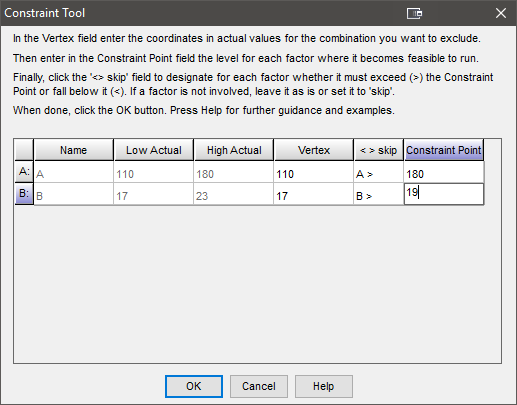1. Click OK and the constraint inequality will be calculated and entered. If there are more constraints they need to be computed one at a time. Use the constraint tool as many times as necessary.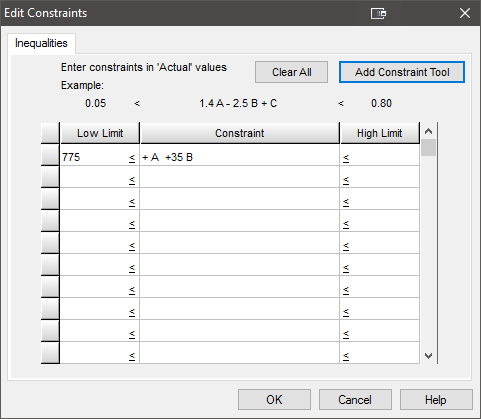1. On the “Design Evaluation”, node look at the contour plot: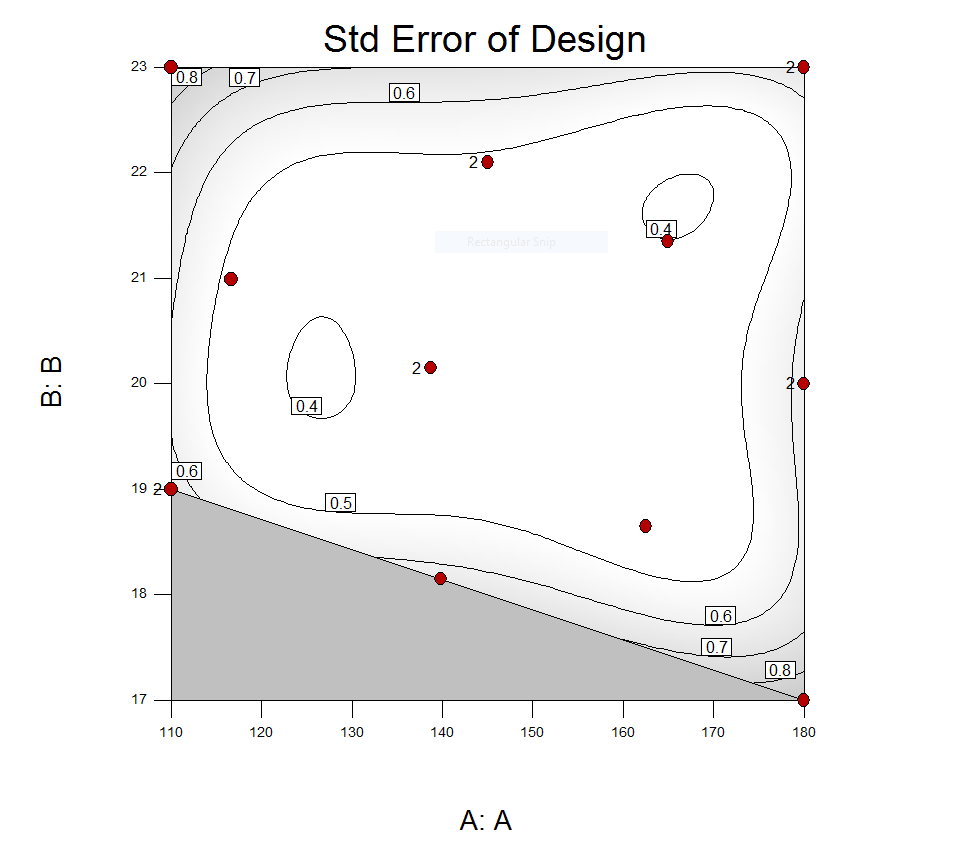### Example #2:¶

1. Define the boundaries using an “Optimal” design: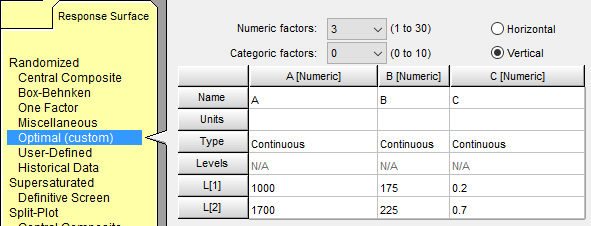There is a constraint at the all high vertex.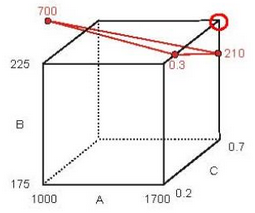1. Click on “Edit Constraints” button and then the “Constraint Tool” button for assistance writing the inequalities.

1. Select the vertex being excluded (in this case the all high vertex) and enter the constraint points. (This constraint excludes two vertices.)

1. When B is 225, and C is 0.7 - A must be no more than 700.

2. When A is 1700, and C is 0.7 - B must be no more than 210.

3. When A is 1700, and B is 225 - C must be no more than 0.3.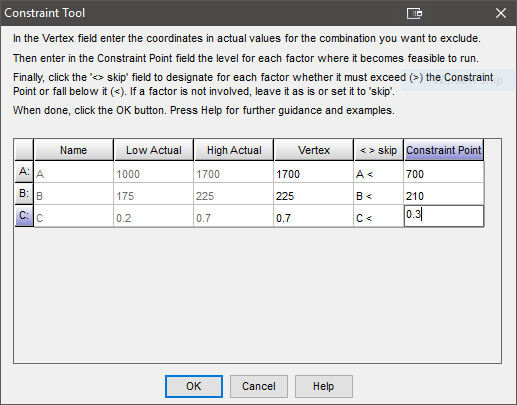1. Click OK after entering the constraint point for each factor. The final OK will produce…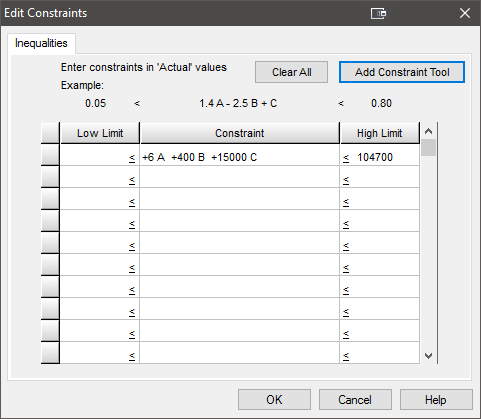### Example #3:¶

1. Define the boundaries using an “Optimal” design: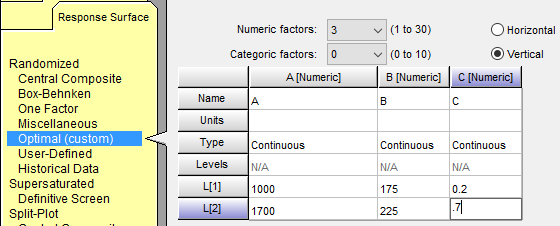There are two constraints on this design. One occurs at the all high vertex, on is at the high, high, low vertex.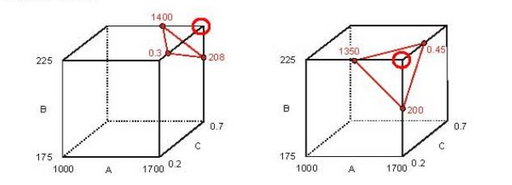1. Click on the “Edit Constraints” button and then the “Constraint Tool” button for assistance writing the inequalities.

1. Select the first vertex, in this case all high, and write the defining statements. For the first constraint enter the Constraint tool…

1. When B is 225, and C is 0.7 - A must be no more than 1400.

2. When A is 1700, and C is 0.7 - B must be no more than 208.

3. When A is 1700, and B is 225 - C must be no more than 0.3.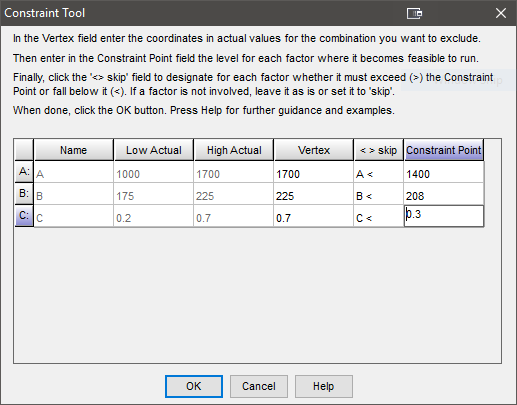Click OK.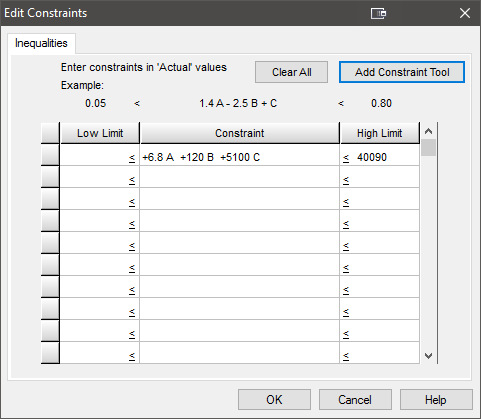For the second constraint, we are at a new vertex on the Constraint tool… Change Vertex C to 0.2, after you do this the “C <” constraint will change to “C >”.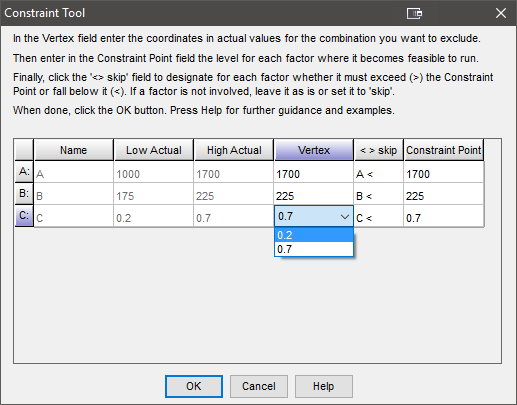Enter the constraint point:

1. When B is 225, and C is 0.2 - A must be no more than 1350.

2. When A is 1700, and C is 0.2 - B must be no more than 200.

3. When A is 1700, and B is 225 - C must be at least 0.45.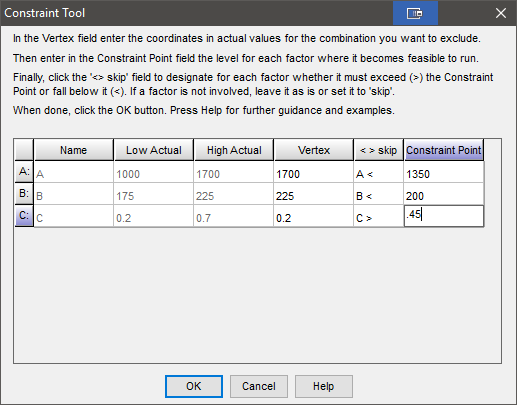1. Click OK after entering the constraint points for each constraint. The final OK will produce…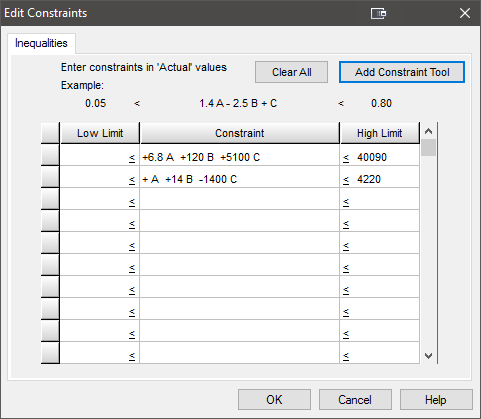### Example #4:¶

1. Define the boundaries using an “Optimal” design: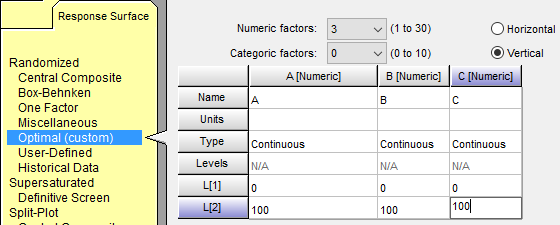There is a single constraint, it is impossible to run experiments when both A and C are at their high levels. B is not involved in this relationship.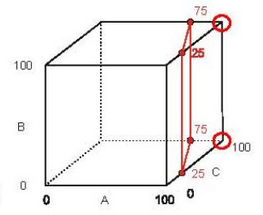1. Click on the “Edit Constraints” button and then the “Constraint Tool” button for assistance writing the inequalities.

1. Define the constraint. For the constraint where A is 100, B is skipped, and C is 100.

1. When C is 100 - A must be no more than 75.

2. B is skipped.

3. When A is 100 - C must be no more than 25.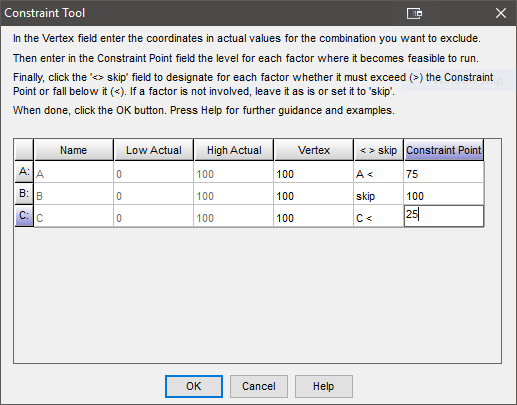1. The final inequality set is…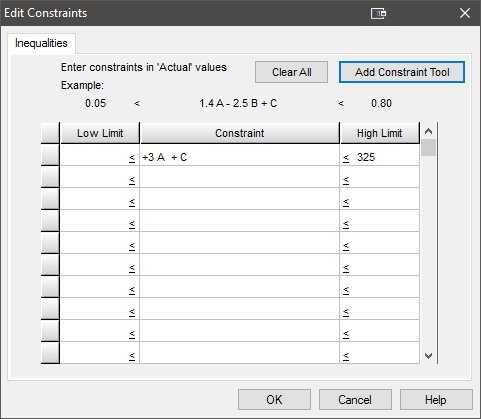### Example #5:¶

1. Define the boundaries using an “Optimal” design: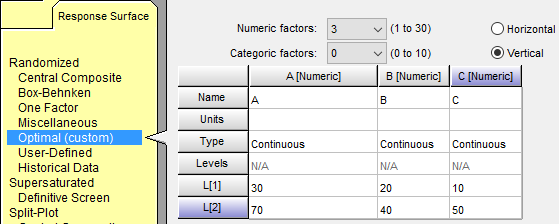There are two constraints on this design, one at the high, low, high vertex, and one at the low, high, low vertex.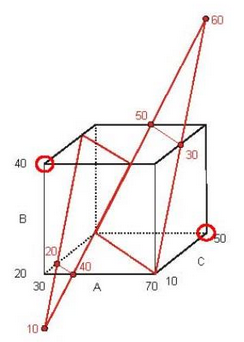1. Click on the “Edit Constraints” button and then the “Constraint Tool” button for assistance writing the inequalities.

1. Define the constraints. For the first constraint where A is 70, B is 20, and C is 50.

1. When B is 20, and C is 50 - A must be no more than 30.

2. When A is 30, and C is 10 - B must be at least 60.

3. When A is 30, and B is 20 - C must be no more than 10.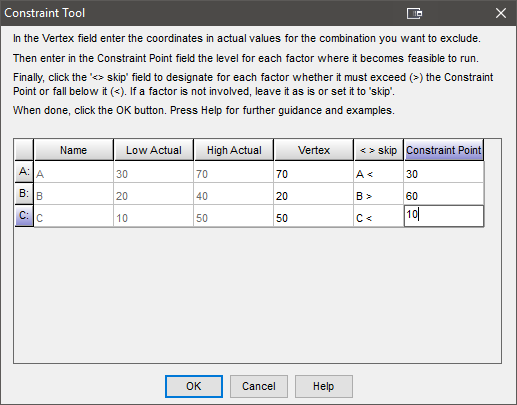For the second constraint where A is 30, B is 40 and C is 10.

1. When B is 40, and C is 10 - A must at least 60.

2. When A is 30, and C is 10 - B must be no more than 10.

3. When A is 30, and B is 40 - C must be at least 40.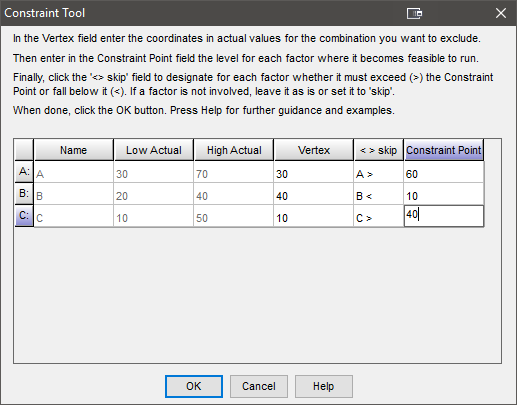1. The final inequality set is…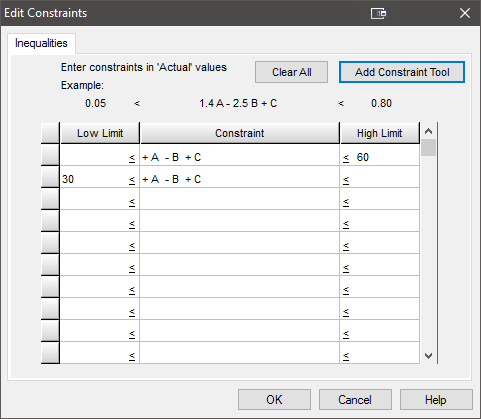1. Which condenses down to…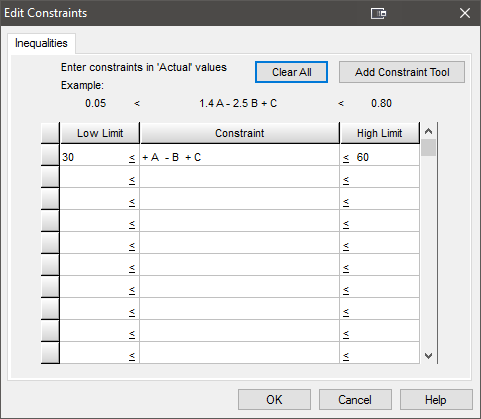Note

Both of these constraints exclude two vertices. Either excluded vertex can be used as a basis for the constraint. Using the other vertices the constraint tool settings can change as can the final inequality, but with a little bit of algebra the inequalities are found to be equivalent. The next example is the same problem but done from the perspective of the other vertices.